# NO5.跑马灯-NEBULA-VSCODEdevcui 2 年前
# nebula-vscode

#### 所属作品集# 1.闪烁 LED 小灯

= 0;”,熄灭 LED 的语句为“led0 = 1;”。按照第 3 章介绍我们重新建立一个 LED 小灯闪烁的工程。程序代码设计如下：

``````#include <STC89xx.h>

// 位声明,将P1-0 管脚声明为LED0
SBIT(LED0, _P1, 0);

void main()
{
// 计数器声明
int i;
while (1)
{
// LED0开启
LED0 = 0x00;
// 计数器延时(计数5000次达到延时效果)
for (i = 0; i < 5000; i++)
;
// LED0关闭
LED0 = 0xFF;
// 计数器延时(计数5000次达到延时效果)
for (i = 0; i < 5000; i++)
;
}
}
``````

# 2.跑马灯

8 个 LED 小灯的硬件电路设计原理图如下图所示：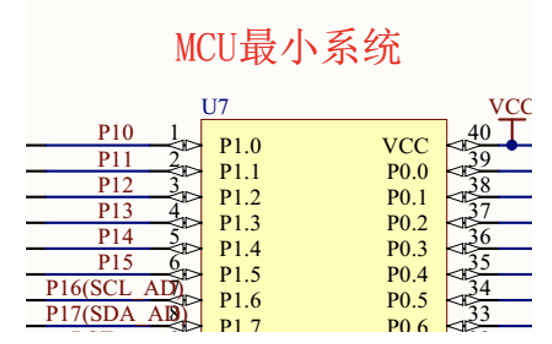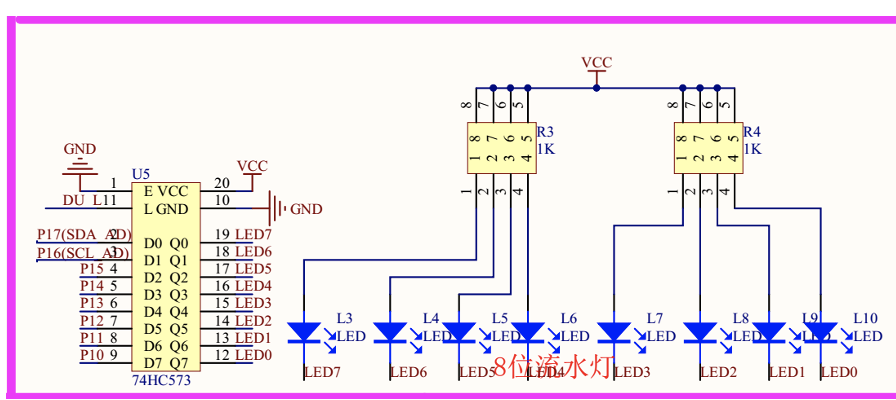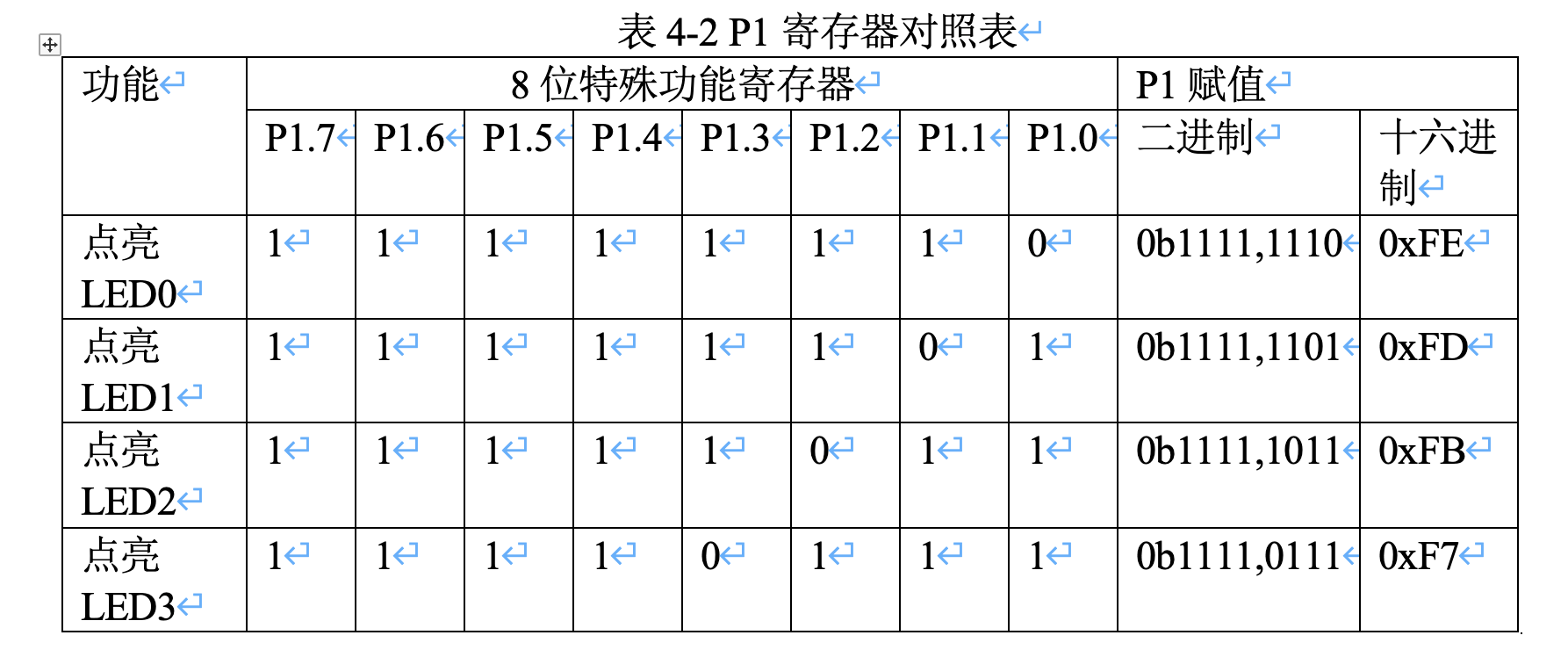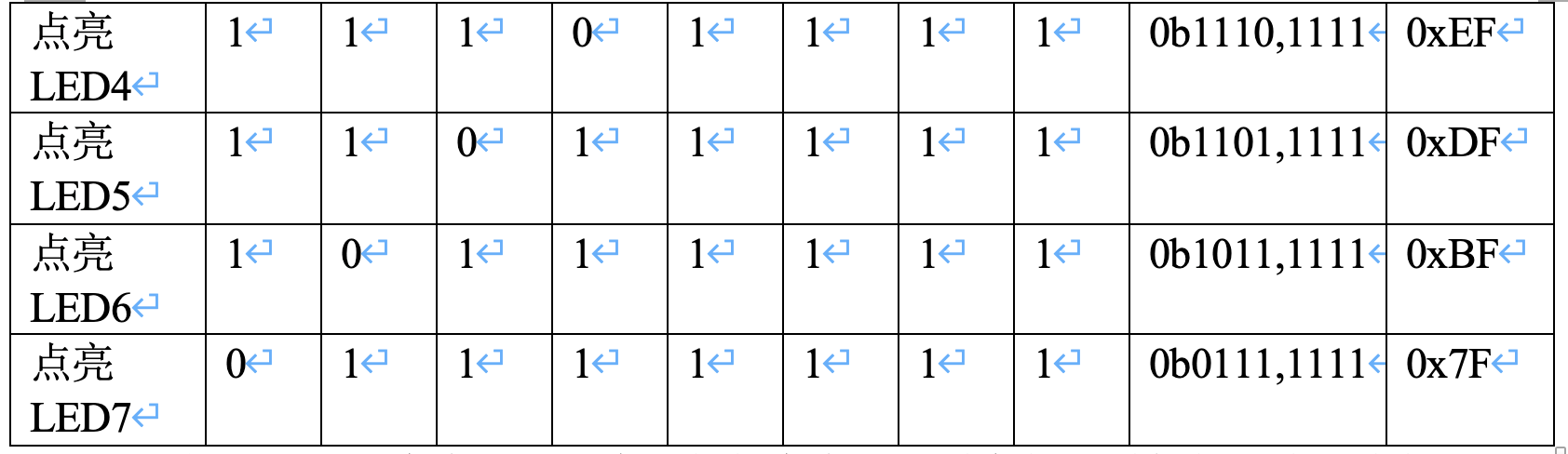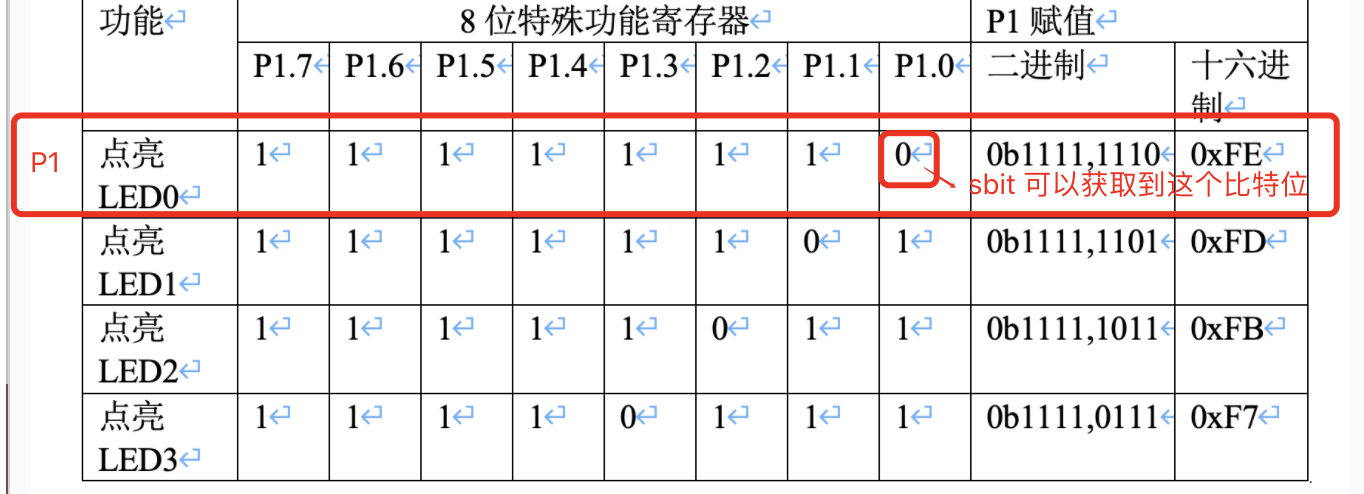## 2.1 代码

``````#include <STC89xx.h>

int i;
int flag = 0;

void main()
{
while (1)
{
P1 = ~(0x01 << flag);
for (i = 0; i < 5000; i++)
;
if (flag > 8)
{
flag = 0;
}
else
{
flag++;
}
}
}
``````

``````<< 是移位的意思
0x01 = 0x0000 0001
0x01 << 0 = 0x0000 0001
0x01 << 1 = 0x0000 0010
0x01 << 2 = 0x0000 0100
``````
``````~是取反的意思，1->0,0->1的意思
~0x01 = ~0x0000 0001 = 0x1111 1110 : 最后一位是P1-0的值，为0所以灯亮了
~0x02 = ~0x0000 0010 = 0x1111 1101 : 倒数第二位是P1-1的值，为0所以灯亮了
~0x03 = ~0x0000 0100 = 0x1111 1011 : 倒数第三为是P1-2的值，为0所以灯亮了
``````

``````if (flag > 8)
{
flag = 0;
}
else
{
flag++;
}
``````

#### 所属作品集